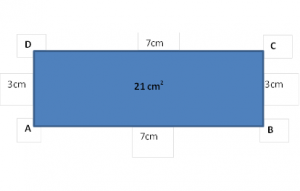# Area and Perimeter Worksheet and Explanation

We’ve structured this information to help children with their education. It is targetted at children in years 5 and 6 and the questions for the worksheet have been stripped from past papers. Having an understanding of area and perimeter is part of the primary school curriculum and children will deal with area and perimeter in both KS1 and KS2.

The Information below will give an overview of the topic and we have included a detailed worksheet with full answers. The worksheet includes 26 area and perimeter questions and is relevant for KS2 pupils approaching their SATS test.

If you are not ready to download the worksheets yet, then read on for some information about area and perimeter. This has provided to introduce the topics covered in the worksheet for those that might be unfamiliar but also as a quick revision tool for those that would like a quick refresher before accessing the worksheet.

## Area and Perimeter

Before looking at area and perimeter it is important that we understand the units of distance first and can convert between them. A line is a one-dimensional shape and is usually measured in cm or m. An area is a two-dimensional shape, meaning it has size in two directions – not just one, like a line, and is measured in cm2 or m2. It is important that children understand these two units and their various forms (e.g. mm, cm and m) as well as how to convert between them (1 m equals 1000 mm).

Area is usually measured in cm2 or m2 is a measure of the size of a surface such as a shape or area of land. It is the space inside a boundary of a flat two-dimensional object such as a circle or triangle.

Perimeter is the distance, measured in cm or m, around the edge of a shape or area of land.

## How to Calculate the Area of a Rectangle

As we can see by the figure below, this rectangle has sides of length 7cm and 3cm. In order to work out the area we have to multiply the height of the rectangle by the length of the rectangle. This gives us the area of the rectangle 21cm2.## How to Calculate the Perimeter of a Rectangle

Using the same rectangle as above we can work out the perimeter of the rectangle by working out how far we would need to travel around the outside of the shape. If we travel from A to B to C to D and back to A (or ABCD) we will travel around the perimeter of the rectangle – a distance of 20 cm. An easier way to calculate in this case is 2(height + length).

Area and perimeter are taught in KS2, with the concepts of distance being taught earlier in stage to provide the basic skills needed to understand area and perimeter. After this children will be introduced to area, starting with simple shapes such as squares before moving onto formula for working out area and perimeter and irregular shapes as they reach year 6.